## selamat datang

semoga blog ini dapat memberi manfaat kepada anda.

## Tuesday, 15 January 2013

### SIMPLE PENDULUM EXPERIMENT

1. Inference :  When the length of a simple pendulum increases, the period of oscillation also increases. // The period of pendulum is affected by the length of the thread.

1. Hypothesis :  The longer the length of a simple pendulum, the longer will be the period of oscillation

3.   Aim :  To find the relationship between the length of a simple pendulum and the period
of oscillation.

4.   Variable :  a) Manipulated variable : Length, l
b) Responding variable  : Period, T.
c)  Fixed variable            : Mass of pendulum bob.

5.       Materials/ Apparatus : Retort stand, pendulum bob, thread, metre rule, stop watch.

FUNCTIONAL DIAGRAM

7. Procedure     :
a)  Set up the apparatus as shown in Figure above.// A small brass or
bob was attached to the thread. The thread was held by a clamp of a
the retort stand.

b) The length of the thread , l  was measured by a metre rule, starting
with 90.0 cm. The bob of the pendulum was displaced and released.

c)  The time for 20 complete oscillations, t was taken using the stop
watch.  Calculate the period of oscillation by using, T = t / 20

d) The experiment was repeated using different lengths such as
80.0 cm. 70.0 cm, 60.0 cm, 50.0 cm and 40.0 cm.

 Length of string, l / cm Time taken for 10 oscillation, t (s) Period of oscillation T T2 (s2 ) t 1 t 2 Average, t 40.0 50.0 60.0 70.0 80.0 90.0 25.2 28.1 31.0 33.5 35.7 38.2 25.1 28.2 31.0 33.6 35.9 37.9 25.2 28.2 31.0 33.6 35.8 38.1 1.26 1.41 1.55 1.68 1.79 1.91 1.59 1.99 2.40 2.82 3.20 3.65

8. Plotting the graph

Notes :

a)   Plotting the graph

·         The graph should be labeled by a heading

·         All axes should be labeled  with quantities and their respective units.

·         The manipulated variable (l) should be plotted on the x-axis while the responding variable (T2 ) should be plotted on the y-axis

·         Odd scales such as 1:3,  1:7 , 1:9 0r 1 :11 should be avoided in plotting  graph.

·         Make sure that the transference of data from the table to the graph is accurate.

·         Draw the best straight line.
- the line that passes through most of the points plotted such that is balanced by the number of points above and below the straight line.

·         make sure that the size  of the graph  is large enough,  which is, not less than half the size of the graph paper or.(  > 8 cm x 10 cm )

10. Discussion / Precaution of the experiment / to improve the accuracy

a)      The bob of the pendulum was displaced with a small  angle
b)      The amplitude of the oscillation  of a simple pendulum  is small.
c)      The simple pendulum oscillate in a vertical plane only.
d)      Switch off the fan to reduce the air resistance

11. Conclusion
The  length of simple pendulum is directly  proportional  to
the square of the period  of oscillation. //

T2 is directly proportional to l  (the straight line graph passing
through the origin)

#### 77 comments:

1.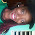how do i get the t^2 divided by s^2?

1.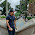what do you mean by that o.O?
s^2 is a unit for t^2... :)

2.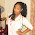This comment has been removed by the author.

3.s= seconds. therefore its seconds squared

4.s= seconds. therefore its seconds squared

5.its T^2 not t^2, therefore its Period (the time taken for one oscillation) and is measured in s^2

6.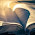Correct.Therefore the period T=t/30 could be this.

7.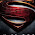Use ur common sense if u have one

8.what is the precaution of this experiment

9.THIS SAVED MY GRADE <3

10.For the t^2 you will square the square the t by 2 .e.g.25.2 for the average of then you square 25.2^2

2.3.4.5.we manufacture physics lab equipment such as, Electrical Instruments, Heat Laboratory Equipment, Mechanics Laboratory Equipment, Measurement Instruments, Meteorology Earth Science Apparatus, Modern Physics Instruments, Optical Instruments, Fluid Mechanics Physics Instruments read more

6.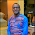how can you compare the theoretical results to the measured experimental?

7.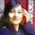We are Physics Apparatus Manufacturers and Physics Apparatus Exporters in India ,Delivered Services to the world Provide Complete solution of laboratory Equipment such as Laboratory Charts,Physics apparatus,Chemistry

8.We are physics lab equipment manufacturer and exporter company in ambala, India since 1954 with a range of products for school, colleges and teaching labs to all countries.

9.i have a doubt in this tabulation for the experiment. the author mentioned T=t/20,(that means time /20 oscilation),But in the tabulation he mentioned for oscilation,i dont understand that,is it a mistake or what?.can you please explain..

1.I think there is a typo in the table. the average time for '10' oscillations in the table has been divided by 20 to give T, so table heading should be for '20' oscillations really

2.yeah, you are right.

10.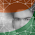How much will g vary at a place and nearby places?

11.12.Ive taken inspiration from this report to conduct my own for my first year lab project at uni, who can i reference this too? i.e. name and date of this report published

13.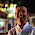14.Why wouldn't angle measurement affect the time?

15.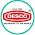I always found worth content here,Would like to visit again.Keep posting interesting articles like this..
PLASTIC LABWARE INDIA

16.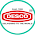This comment has been removed by the author.

17.why is it necessary to record time with respect to the reference mark at the equilibrium position of the bob?

1.Can someonw please help Me with the same question????

18.why is it necessary to add the radius of the bob to the length of the string to know the length of the pendulum??

1.you need to treat the bob as a mass at a point. the point we use is the centre of mass of the bob, and is at a distance of length of string + radius of bob away from the point which remains stationary at top of pendulum

19.how do you calculate period of oscillation?

1.pick a point on the path of the bob - as it passes its lowest point in the centre is usually easiest. Time how long it takes for the bob to pass this point 20 times going in the same direction eg left to right. divide that time by 20 to get the time of one oscillation which is centre to right, all the way to left and then back to centre

20.uncertainity for g is not included

21.Why do we use 20 oscillations rather than one oscillation?

22.This comment has been removed by the author.

23.How can i get T^2 in the table.

24.thank you very much for the clear methods, maybe add a risk assessment in your future experiment posts? :)

25.I want different question of bob pendulum and how to solve

26.How do you find the gradient of the slope/best fit line?

27.This comment has been removed by the author.

28.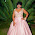can somebody please explain the hypothesis for me???

29.How does mass affect the oscillation of a body

30.I m searching for acceleration due to gravity using simple pendulum

31.Thank You very much! This helped me a lot on my science project.

32.Hi again! Just wanna ask about something. What will the background info be since my teacher wants to know that

33.Hey guys, if you are planning to set up a science laboratory, then you should check out the Atico Export, it is an online store of science industries. From this site you can order your equipment online by providing your specifications.

34.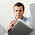This comment has been removed by the author.

35.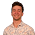Why is it important that the angle used is 10 degrees?

36.This comment has been removed by the author.

37.This is really an interesting blog as it focuses on the very important topic. i came to know about so many things or tips.

Educational Lab Instruments Exporters

38.thanks this is much helpful to me on my lap report

39.Pls state the value of the constant you deduced and the estimated errors I don't get this

40.I really found this site interesting.........Many thanks

41.How to find g from graph

42.What is the need of several oscillations instead of few

43.How to find slope from the graph?

44.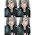sabung ayam laga bangkok taji pisau

45.What if i consider my g to be 4 pie squared divided by the gradient of the graph of T squared against l?

46.What if i consider my g to be 4 pie squared divided by the gradient of the graph of T squared against l?

47.48.Is t2 proportional to l if it's close to a straight line?

49.This comment has been removed by the author.

50.Amazing post!! A huge range of interested topics are here to improve an individual knowledge. Read more about Physics Lab Instruments Manufacturer

51.52.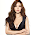53.54.Nice explanation

55.56.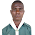Thanks for your contribution

57.Nyc but how can we calculate length of second's pendulum

58.Tq soo much . May god bless yoh

59.pls how do u take your time readings? it minute:second
or it just seconds
because when I did the practical for length 50cm my I got 0.26

60.Thanks for sharing this useful information. I am collecting more details about Physics Lab Equipments Supplier. If you have relevant data then kindly keep sharing.

61.what would be the comparison if we were to compare between compound and simple pendulum

62.Why is the graph negative when plotted h against T². And what's the cause of the graph not passing through the origin